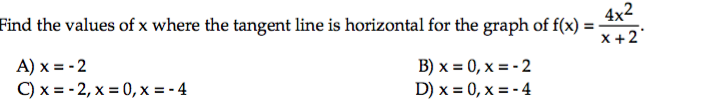# 1. Cheraw Auto Repair believes that it will need new equipment in 10 years. The equipment will co...

1. Cheraw Auto Repair believes that it will need new equipment in 10 years. The equipment will cost 11) \$26,000. What lump sum should be invested today at 8% compounded semiannually, to yield \$26,000?

2.4x2 x 2 Find the values of x where the tangent line is horizontal for the graph of f(x)- A)x=-2 C)x=-2, x=0, x=-4 B)x=0,x=-2 D)x=0,x=-4

Semi annual interest=(8/2)=4%=0.04

Future value (FV)=Present Value (PV)*((1+i)^N)

i=Interest per period=0.04

N=Number of Periods=10*2=20

Future Value (FV)=\$26000

Lump sum to be invested today=PV=FV/((1+i)^N)=26000/(1.04^20)= \$11,866.06

 Lumpsum to be invested today \$11,866.06
##### Add Answer of: 1. Cheraw Auto Repair believes that it will need new equipment in 10 years. The equipment will co...
More Homework Help Questions Additional questions in this topic.

• #### You are the auditor of Jexel, an auto air-conditioner service and repair company, and you have de...

Need Online Homework Help?